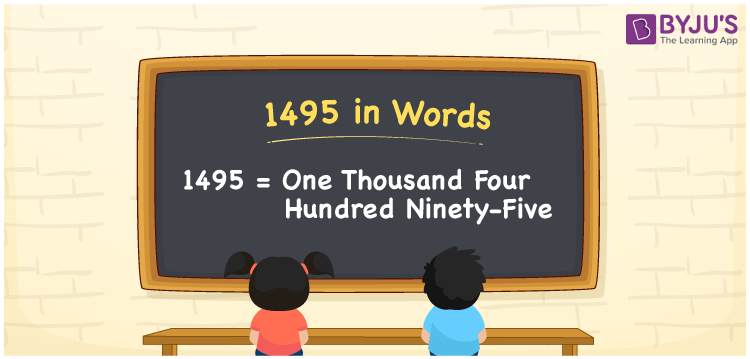# 1495 in Words

1495 in words is written as One Thousand Four Hundred Ninety-Five. For example, Siya purchased a handbag worth Rs. 1495, then you can express the same sentence in words as “Siya purchased a handbag worth Rupees One Thousand Four Hundred Ninety-Five”. 1495 is a cardinal number since it denotes the quantity or value of something. This article explains the simple tricks used in converting the numbers to words using a place value chart. Also, learn the spelling of the number 1495 in English words here.

 1495 in Words One Thousand Four Hundred Ninety-Five One Thousand Four Hundred Ninety-Five in numerical form 1495

## 1495 in English Words

We can write the numbers in words with the help of the letters present in the English alphabet. Therefore, we can spell the number 1495 in English words as One Thousand Four Hundred Ninety-Five.## How to Write 1495 in Words?

We can convert the number 1495 to words using a 4 column table as it is a four-digit number. The table mentioned below depicts the place value chart for the number 1495.

 Thousands Hundreds Tens Ones 1 4 9 5

Hence, we can write the expanded form as:

1 x Thousand + 4 x Hundred + 9 x Ten + 5 x One

= 1 x 1000 + 4 x 100 + 9 x 10 + 5 x 1

= 1000 + 400 + 90 + 5

= 1495

= One Thousand Four Hundred Ninety-Five

Hence, 1495 in words is written as One Thousand Four Hundred Ninety-Five

Interesting way of writing 1495 in words

1 = One

14 = Fourteen

149 = One Hundred and Forty-Nine

1495 = One Thousand Four Hundred Ninety-Five

Thus, the word form of the number 1495 is One Thousand Four Hundred Ninety-Five

1495 is a natural number that precedes 1496 and succeeds 1494

• 1495 in words – One Thousand Four Hundred Ninety-Five
• Is 1495 an odd number? – Yes
• Is 1495 an even number? – No
• Is 1495 a perfect square number? – No
• Is 1495 a perfect cube number? – No
• Is 1495 a prime number? – No
• Is 1495 a composite number? – Yes

## Frequently Asked Questions on 1495 in Words

Q1

### How to write 1495 in words?

1495 in words is written as One Thousand Four Hundred Ninety-Five.
Q2

### What is the value of 1000 + 495 in words?

1000 + 495 = 1495. Hence, the value of 1000 + 495 in words is One Thousand Four Hundred Ninety-Five.
Q3

### Is 1495 an odd number?

Yes, the number 1495 is an odd number.